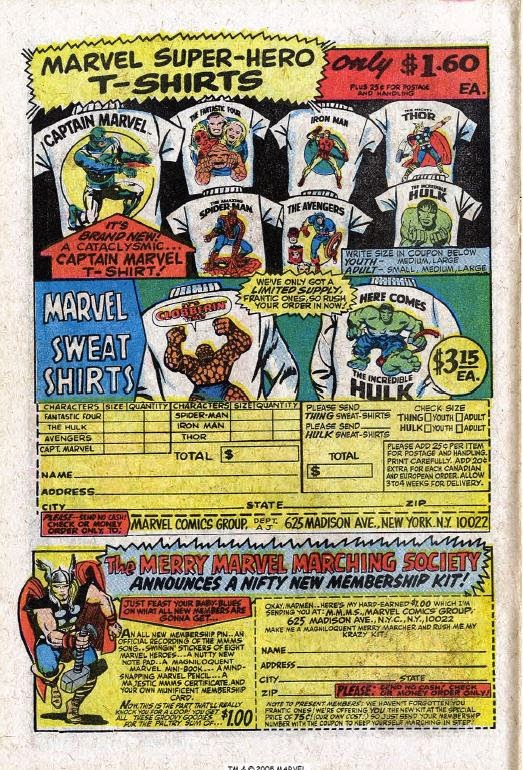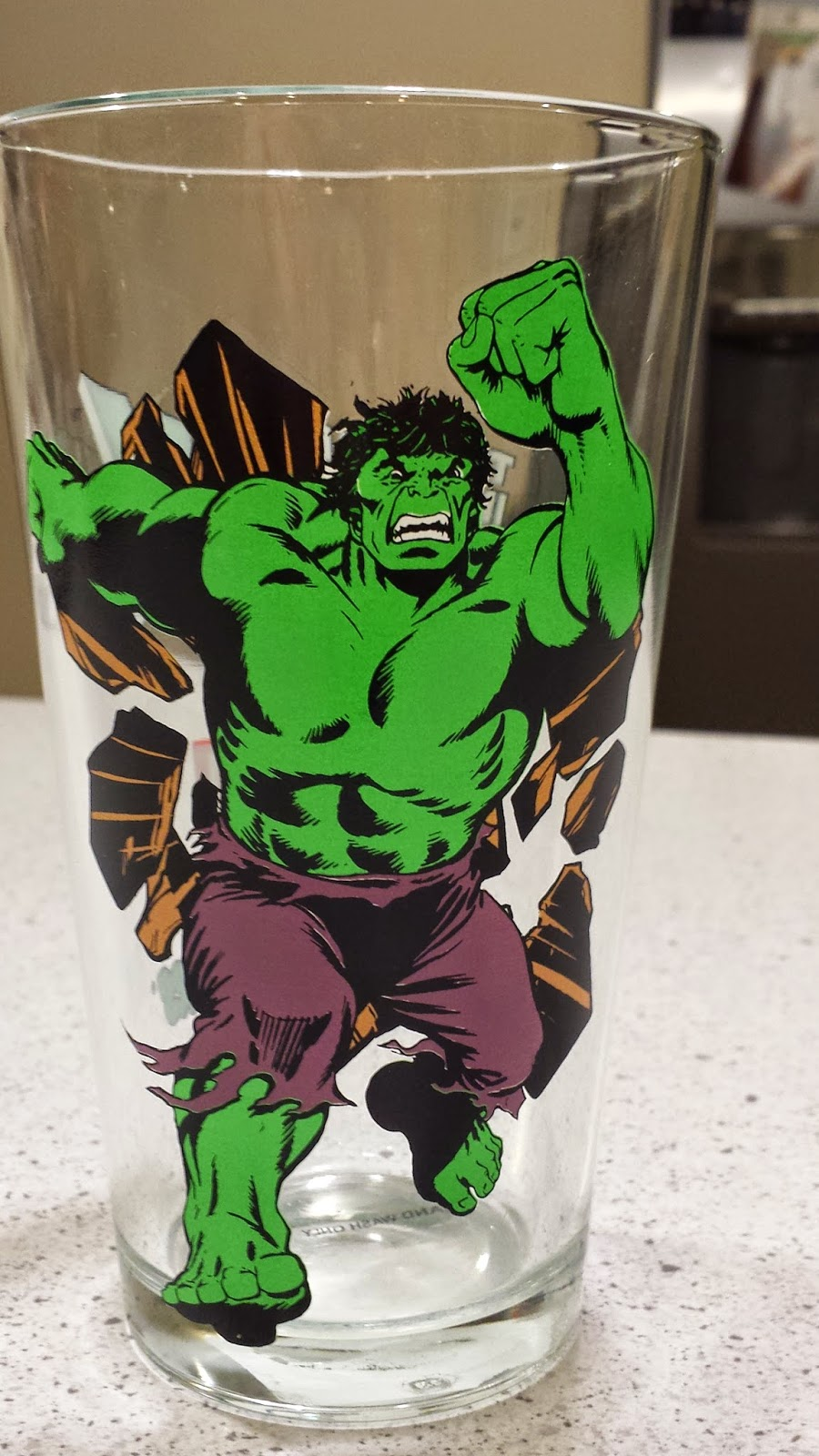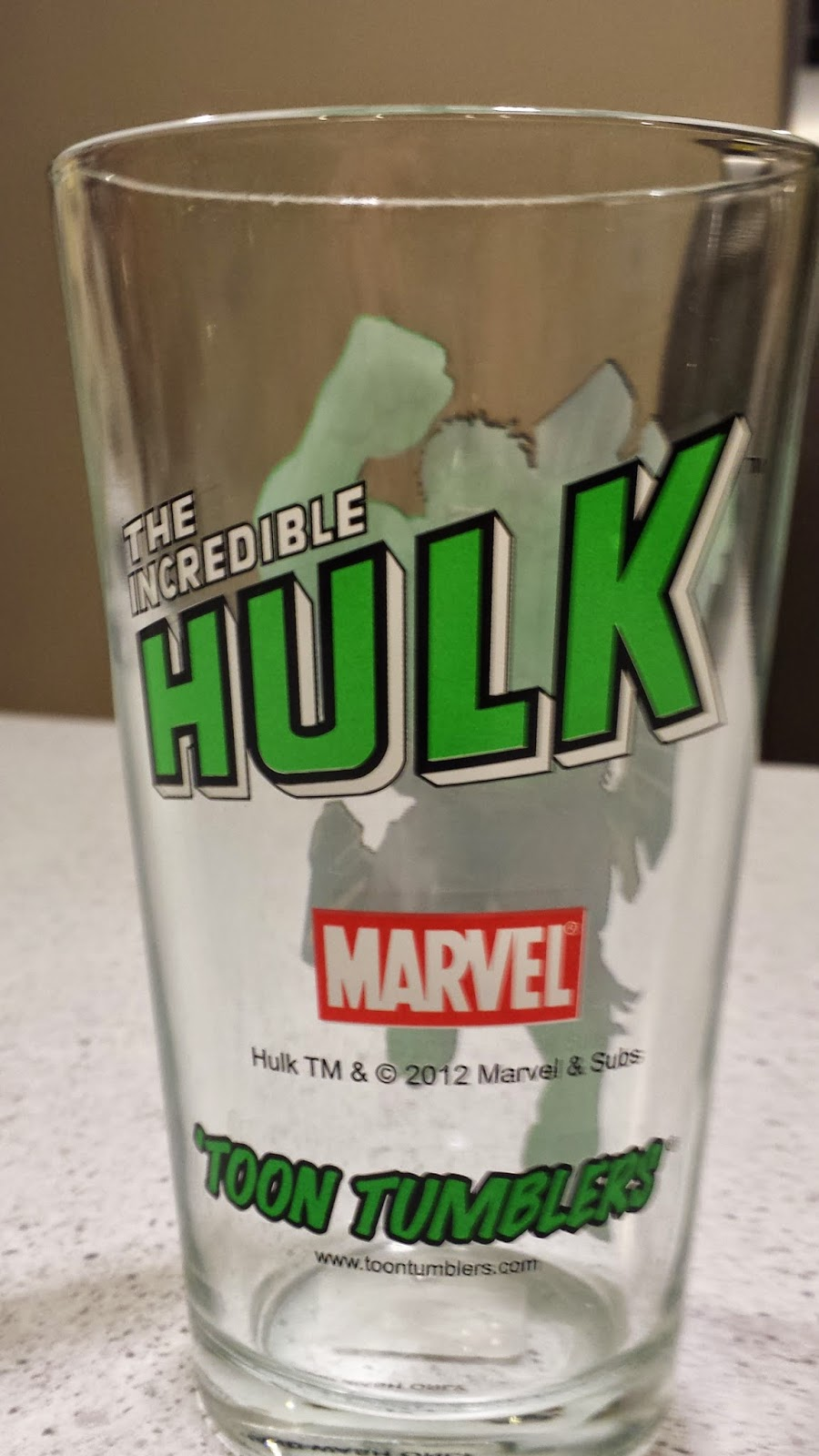## Hey, Mom! The Explanation.

Here's the permanent dedicated link to my first Hey, Mom! post and the explanation of the feature it contains.

## Sunday, February 1, 2015

### Weekly Comics for 1408.13

Weekly Comics for 1408.13

I have always liked the Hulk.

My father liked the Hulk, too. Maybe it was the science fiction flavors of some of those early 1960s sagas. Or maybe it was just the new take on the Jekyll-Hyde split personality of the good gamma irradiated doctor, all that good pathos, the suffering. David Bruce Banner finds himself doomed to a solitary and nomadic existence, never able to have a normal life whereas the Hulk seems a simpleton but is actually complex. Is he a good guy or a bad guy? What does he want? And how many ways are there to position him against adversaries in which he ends up being a force for good.

The Incredible Hulk was one of our favorite comics to read in those bed times of my long ago youth along with Fantastic Four and Batman. The Hulk had so much to teach us about the internal conflict of mankind, the desire to be left alone, the need for peace and serenity, something denied the Hulk as trouble and battles would always find him. After all, in those early days, it was a style guide requirement that each comic feature at least one battle.

But there are so many iterations of Bruce Banner-Hulk now as well as other Hulks (though Hulk, now Doc Green, is eliminating the other gamma powered beings in the current story line) the question of loving the Hulk causes consideration of a fractal. Which Hulk?

The Hulk has been re-imagined many times. He is in the middle of that re-imagining now as "Doc Green," in which he has Banner's intelligence and the Hulk's strength, but has supposedly eliminated Banner. Doc Green is also more cunning and ruthless that previous smart Hulks. Though this new version of the Hulk keeps the character fresh, there's a loss to the pathos for the eternal wandering monster, the misunderstood creature, who continually finds himself helping a world that hates and fears him.

That's all for now in the front matter. My blog output has slowed. I need to pick it up.

+=+=+=+=+=+=+=+=+=+=+=+=+=+=+=+=+=+=+=+=+=+=+=+=+=+=+=+=+=+=+=+=+=+=+=++=+=+=+=+=+=+=+=+=+=+=+=+=+=+=+=+=+=+=+=+=+=+=+=+=+=+=+=+=+=+=+=+=+=+=++=+=+=+=+=+=+=+=+=+=+=+=+=+=+=+=+=+=+=+=+=+=+=+=+=+=+=+=+=+=+=+=+=+=+=++=+=+=+=+=+=+=+=+=+=+=+=+=+=+=+=+=+=+=+=+=+=+=+=+=+=+=+=+=+=+=+=+=+=+=++=+=+=+=+=+=+=+=+=+=+=+=+=+=+=+=+=+=+=+=+=+=+=+=+=+=+=+=+=+=+=+=+=+=+=++=+=+
SPOILERS SPOILERS SPOILERS SPOILERS SPOILERS
SPOILERS SPOILERS SPOILERS SPOILERS SPOILERS
SPOILERS SPOILERS SPOILERS SPOILERS SPOILERS
SPOILERS SPOILERS SPOILERS SPOILERS SPOILERS
RE: SPOILERS: I don't have to explain that you read at your own risk, right?
=+=+=+=+=+=+=+=+=+=+=+=+=+=+=+=+=+=+=+=+=+=+=+=+=+=+=+=+=+=+=+=+=+=++=+=+=+=+=+=+=+=+=+=+=+=+=+=+=+=+=+=+=+=+=+=+=+=+=+=+=+=+=+=+=+=+=+=+=++=+=+

CBR FOR AUGUST 13 2014

IGN FOR AUGUST 13 2014

WEEKLY COMICS LISTSo, given the comments above, I am Hulk fan, which reminds me of the classic Marvel maniacs T-shirts sold via in-house ads in the comics of my youth, such as this one to the left.

I wondered if the old style T-shirts are still for sale somewhere. I do not own a T-shirt for the Hulk, and I would like some of these others, too, like Captain Marvel, the Thing, and Thor. I wanted these shirts when I was a kid, especially the Fantastic Four T-shirt, but I never owned any of them.

Look at those prices!! \$1.60? \$3.15? Those were "expensive" shirts. Remember that comics are 12 cents at this time. This ad is from a comic in 1969. I was seven years old.

Hulk may have been feared and hated by those in the world of the comics, but he was much loved by those READING the comics. He is still loved today, though maybe less so in his current incarnation.

There are images posted of those old T-shirts, but I have yet to find anyone selling them.

Ah... but this ad brings back memories. I spent a lot of time staring at it. I can still be sucked in by it.All this Hulk content made me buy a Hulk drinking glass, though the item will not appear in my lists for many weeks as it is 1501.30 as I type this, and I just fetched comics from 1501.28 today, Friday.

As I cobble together thoughts on comics, just to be busy doing some writing of words rather than C code, I am reminded that I had this bright idea to write about comic books many years ago as a young boy, about seventh grade, when I first was given a typewriter. But this is a story for another time.

Back to the HULK. I am disappointed to see that this week's issue of the Hulk received such median reviews from the comic book "Bloggonistirati" (I just made up that word).Here's a guy who gave it a 10/10 at COMIC BOOKED BUT other reviews, much lower, like this one at Newsarama, evened out the average to 7.6 for Hulk #005.

For me the book gets extra points for Mark Bagley's art, which I have always loved despite his angular figures reminiscent of Cubism.

Despite my comments earlier, this new iteration of the Hulk is very fun. The great thing about comics is that new iterations do not OVERWRITE the old ones. The original Hulk (the grey more villain like one) and the main green behemoth best known for the late 1960s and 1970s work, especially my favorite the Rampaging Hulk magazine with its dynamic SF story line, still exist and may be as close as a digital download away if not a trip to the local comic book shop.

Hey, when I was a kid, there were no comic book shops in Kalamazoo let alone prevalently nationwide.

Despite my comments about the Hulk, the ongoing destruction of the Fantastic Four takes top spot this week, and the penultimate Original Sin takes the third slot, mostly on the strength of Mike Deodato's art and the characters involved, namely Doctor Strange and The Black Panther, two of my favorites.

Now looking at this list, I am puzzled as to why the excellent Batman comic, that I have written of frequently hereabouts, feel so low. But I promised I would not feature every cover nor write long diatribes about all comics in this week or this week in general. But I do think that if I did not re-order these comics as I read them, I surely would re-order them now.

Other comics of excellence like Sex Criminals and The Walking Dead, I have discussed before and will again. So that's all for now.

I will add that the revenge of the Black Cat featured in Amazing Spider-Man: Original Sin #5 was just as good as the Superior Spider-Man issue in which his Doc Ock possessed self punches her out and has her arrested.

If nothing else, each of the big two companies is working hard to keep the stories and characters in these franchises fresh and dynamic.

Hmmmmmmmmmmmm ... second use of "dynamic" today. Time to stop.

RANKED COMICS from AUGUST 13, 2014

Fantastic Four #8
Hulk #005
Original Sin #7 of 8
The Walking Dead #130
Sex Criminals #7
Amazing Spider-Man: Original Sin #5
All New X-men #030
Justice League United #4
Starlight #5
Batman #34
Batgirl #34
Red Sonja #11
Captain America #023
Spider-Man 2099 #002
Avengers World #011
Captain Marvel #006

BACK LOG

The New 52: Futures End #15
Batman Eternal #19
Superman/ Wonder Woman: Doomed #11
World's Finest: Huntress and Power Girl #26

SPECIAL PURCHASE

Locus #643 Vol. 73 No. 2

+=+=+=+=+=+=+=+=+=+=+=+=+=+=+=+=+=+=+=+=+=+=+=+=+=+=+=+=+=+=+=+=+=+=+=++=+=+=+=+=+=+=+=+=+=+=+=+=+=+=+=+=+=+=+=+=+=+=+=+=+=+=+=+=+=+=+=+=+=+=++=+=+=+=+=+=+=+=+=+=+=+=+=+=+=+=+=+=+=+=+=+=+=+=+=+=+=+=+=+=+=+=+=+=+=++=+=+=+=+=+=+=+=+=+=+=+=+=+=+=+=+=+=+=+=+=+=+=+=+=+=+=+=+=+=+=+=+=+=+=++=+=+=+=+=+=+=+=+=+=+=+=+=+=+=+=+=+=+=+=+=+=+=+=+=+=+=+=+=+=+=+=+=+=+=++=+=+

COVER GALLERY
- Bloggery committed by chris tower - 1502.01 - 15:47